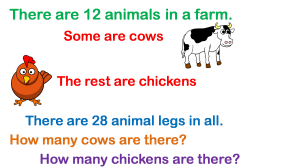﻿ MatheMagis Singapore Maths » Blog Archive » Can the bar-model be used to solve all math word problems?

### Can the bar-model be used to solve all math word problems?No. Some problems do not lend themselves to bar-modeling. Singapore Math teaches various strategies, called heuristics, for solving these kinds of so-called non-routine word problems. There are about nine heuristics used in Singapore Math. These strategies are taught as early as Primary 1 and spirals through Primary 6.

How does a student know when to use the bar-model and when to use heuristics? There are no hard and fast rules on when to use a specific strategy in problem solving. However, exposure to a variety of word problems, hours of practice and proper teaching methodology help, of course. In the end, the goal of Singapore math is to arm the students with various strategies to solve a variety of word problems and to develop analytical skills to decide which strategy is most applicable in a given problem or situation.